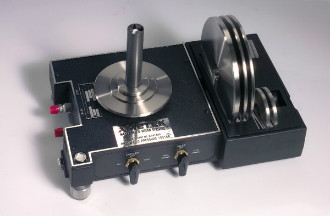# Fundamentals of Pressure Measurement

## Fundamentals of Pressure Measurement

Pressure, by definition, is a derived para-meter. One cannot create an artifact of one pound per square inch or any other measure of pressure. Pressure is derived by the combination of a mass measurement imposed upon an area. It is commonly expressed in terms of pounds force or per unit area (Pounds per square inch). Pressure can also be expressed in terms of the height of a liquid column (Inches of water or millimeters of mercury) that produces the same pressure at its base.

### Gauge Versus Absolute PressurePressure measurements are always expressed as the difference between the measured pressure and some base pressure. Gauge pressure is the pressure measured from, or in addition to, atmospheric pressure. Gauge pressure is normally expressed in terms such as PSIG or pounds per square inch gauge. Absolute pressure is measured from a base of zero pressure and is expressed as PSIA or pounds per square inch absolute. Negative pressures such as vacuum are expressed as the difference between atmospheric pressure and the measured pressure.

Vacuum or negative pressures are normally expressed as inches or millimeters of mercury or water vacuum.

### Nominal Versus Actual Pressure

The accuracy of the generated pressure is measured as the difference between the actual pressure produced by the pressure standard and a standard pressure to be used for the calibration of a secondary standard pressure measuring instrument. AMETEK deadweight testers are referred to “Nominal” or even unit pressures, such as 1000, 2000, 3000 PSIG etc.

Pressure standards manufactured by other manufacturers, Ruska, D.H., refer the output pressure to the actual output pressure as stated on the certification or computed by an equation included within the certification. AMETEK accuracy is therefore the difference between the output pressure and the nominal pressure stated in percentage of the reading.

AMETEK further states the ability of the instrument to repeat identical pressures with identical weights and piston as the percentage of “Repeatability”. Ruska, D&H accuracy as stated is the statistical ability of the instrument to repeat the identical pressure with the same weights and piston. This is comparable to AMETEK’s stated percentage for instrument repeatability.

### Units of Pressure Measurement

Pressure is measured in several different units depending upon the application and the country in which the measurement is taken. Within the United States, the most common unit of measure is Pounds (Force) per Square Inch, for low pressure measurements a measure of Inches of Water Gauge and for vacuum Millimeters of Mercury Vacuum. The official unit of pressure measurement within United States is the Pascal which is defined as Newton per square meter. A detailed listing of the various measures of pressure and the equivalent pressure in pounds per square inch is as follows:

### Low Pressure

Inches of Water Gauge (20oC) =

0.036063 PSI (ISA RP 2.1)

Inches of Water Gauge (60oF) =

0.036092 PSI (AGA Report 3)

Inches of Water Gauge (4oC) = 0.036126 PSI

Millimeters of Water Gauge (20oC)= 0.0014198 PSI

Millimeters of Water Gauge (4oC)= 0.00142228 PSI

Vacuum

Inch of Mercury (0oC)= 0.49114999 PSI

Torr = 1 Millimeter of Mercury (0oC) = 0.019718 PSI

Millimeters of Mercury (20oC) = 0.019266878 PSI

Pressure

Pascal = 1 Newton/Meter2 = 0.0001450377 PSI

Bar = 100 Kilopascal = 14.50377 PSI

Kilogram (Force) per Square Meter = 14.22334 PSI

### Units of Mass MeasurementThe term mass as used in the mathematical expressions for pressure is understood to be the true mass or the mass value that would be measured in a vacuum. Although this is the value required for the pressure equation, many different methods have been used by both Deadweight Tester manufacturers and calibration facilities. These methods fall into two categories: true mass and apparent mass versus some material of a different stated density. Typical materials and conditions for apparent mass are brass with a density of 8400 kg/m3 and stainless steel at 8000 kg/m3, both measured at 20ºC.

### Gravity

The term force that is used within the deadweight tester mathematical expression for pressure is defined as the mathematical product of the true mass and the local gravity. The total variation due to gravity over the surface of the earth can vary as much as 0.5%. Acceleration due to gravity can be calculated as follows:

g = 980.6160 (1 – 0.0026373 cos 2ö + 0.0000059 cos2ö)

Where ö is the latitude at sea level and

g1 = gö- .0003086 h + .0001118 (h -h1)

Where h1 is the elevation, in meters, of the general terrain for a radius of 70 km and h, in meters, is the elevation of the location above sea level.

An exact measurement of a specific location can be achieved by the conduct of a gravinometric survey. An estimate of local gravity for continental United States locations is available at nominal cost from the United States Department of Commerce, National Ocean Survey.

### Primary and Secondary Pressure Standards

Primary Pressure standards must be directly traceable to the physical standards of length and mass, and any errors must either be eliminated or evaluated. Within the deadweight tester the area of the piston and cylinder or the ball and nozzle can be measured and directly traceable to the physical standard of length. The weights can be measured directly traceable to the physical standard of mass. The only other pressure measurement device that fulfills this definition of primary is the U tube manometer wherein the column difference is traceable to length and the fluid density is traceable to both mass and length.

All other devices for measuring pressure, regardless of accuracy, uncertainty, etc. are considered as secondary. This includes electronic, quartz tube, vibrating cylinder, etc. type pressure measuring instruments.

Source: AMETEK